Courses

# Mechanical Oscillations (Part -1) - Oscillations and Waves, Irodov JEE Notes | EduRev

## JEE : Mechanical Oscillations (Part -1) - Oscillations and Waves, Irodov JEE Notes | EduRev

The document Mechanical Oscillations (Part -1) - Oscillations and Waves, Irodov JEE Notes | EduRev is a part of the JEE Course I. E. Irodov Solutions for Physics Class 11 & Class 12.
All you need of JEE at this link: JEE

Q. 1. A point oscillates along the x axis according to the law x = a cos (ωt — n/4). Draw the approximate plots
(a) of displacement x, velocity projection vx, and acceleration projection wx  as functions of time t;
(b) velocity projection vx  and acceleration projection wx  as functions of the coordinate x.

Ans. 1. (a) Given, x =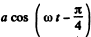So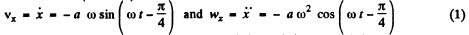On-the basis of obtained expressions plots x(t) , vx(t) and wx(t) can be drawn as shown in the answersheet, (of the problem book ).

(b) From Eqn (1)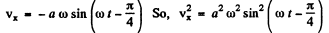(2)

But from the law x = a cos (ωt - π/4) , so, x 2 = a2 cos2 (ωt - π/4)

or, cos (ωt - π/4) - X2/ = 2 or sin2 (ωt - 3t/4) =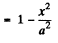(3)

Using (3) in (2),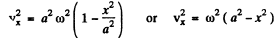(4)

Again from Eqn (4), wx = -aω2cos (ωt - π/4) = -ω2x

Q. 2. A point moves along the x axis according to the law x = a sin2(ωt — π/4). Find: (a) the amplitude and period of oscillations; draw the plot x (t); (b) the velocity projection vx  as a function of the coordinate x; draw the plot vx  (x).

Ans. 2. (a) From the motion law of the particle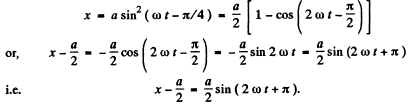(1)

Now compairing this equation with the general equation of harmonic oscillations : X - A sin(ωot+a) Amplitude, A = a/2 and angular frequency, ω0 - 2ω.

Thus the period of one full oscillation,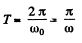(b) Differentiating Eqn (1) w.r.t. time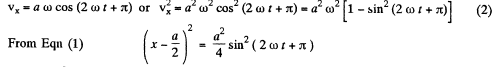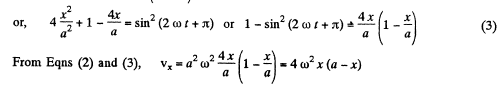Plot of vx (x) is as shown in the answersheet.

Q. 3.A particle performs harmonic oscillations along the x axis about the equilibrium position x = 0. The oscillation frequency is  ω = 4.00 s-1 . At a certain moment of time the particle has a coor- dinate xo  = 25.0 cm and its velocity is equal to vx0  = 100 cm/s. Find the coordinate x and the velocity vx of the particle t = 2.40s after that moment.

Ans. 3 Let the general equation of S.H.M. be
h = a cos (ωt + α)
So, vx = - a ω sin (ωt + α)
Let us assume that at t = 0 , x = h0 and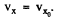Thus from Eqns (1) and (2) for t = 0, h0 = a cos  α, and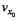= - a ωsin α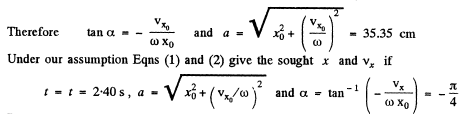Putting all the given numerical values, we get :

x = - 29 cm and vx = - 81 cm/s

Q. 4.Find the angular frequency and the amplitude of harmonic oscillations of a particle if at distances x and x2  from the equilibrium position its velocity equals v1 a nd v2  respectively.

Ans.  From the Eqn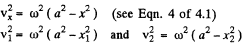Solving these Eqns simultaneously, we get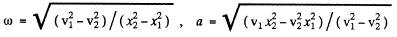Q. 5. A point performs harmonic oscillations along a straight line with a period T = 0.60 s and an amplitude a = 10.0 cm. Find the mean velocity of the point averaged over the time interval during which it travels a distance a/2, starting from
(a) the extreme position;
(b) the equilibrium position.

Ans.  (a) When a particle starts from an extreme position, it is useful to write the motion law as x = a cos ωt             (1)

(However x is the displacement from the equlibrium position)

It tx be the time to cover the distence a/2 then from (1)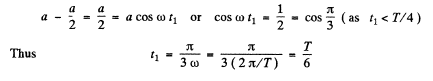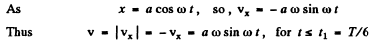Hence sought mean velocity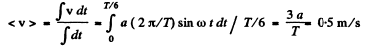(b) In this case, it is easier to write the motion law in the form :

x = a sin ωt                       (2)

If t2 be the time to cover the distance a/2, then from Eqn (2)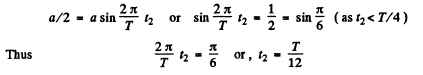Differentiating Eqn (2) w.r.t time, we get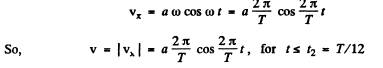Hence the sought mean velocity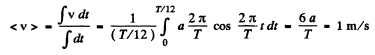Q. 6. At the moment t = 0 a point starts oscillating along the x axis according to the law x = a sin ωt. Find:
(a) the mean value of its velocity vector projection (vs);
(b) the modulus of the mean velocity vector |(v)| ;
(c) the mean value of the velocity modulus (v) averaged over 3/8 of the period after the start.

Ans. (a) As x = a sin ωt                  so,   vx = aω cos ωt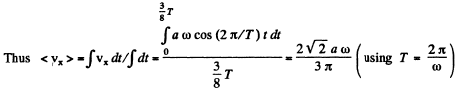(b) In accordance with the problem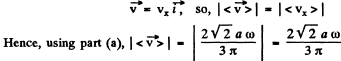(c) We have got, vx = a ω cos ωt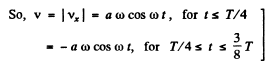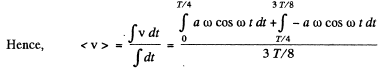Using ω = 2 ω/T , and on evaluating the integral we get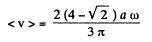Q. 7. A particle moves along the x axis according to the law x = a cos ωt. Find the distance that the particle covers during the time interval from t = 0 to t.

Ans. From the motion law, x = a cos ωt„ it is obvious that the time taken to cover the distance equal to the amplitude (a), starting from extreme position equals T/4.
Now one can write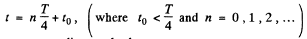As the particle moves according to the law, x .= a cos ωt, so at n = 1,3,5 ... or for odd n values it passes through the mean positon and for even numbers of n it comes to an extreme position (if t0 = 0).
Case (1) when n is an odd number : In this case, from the equation

x = x a sin ωt, if the t is counted from nT/4 and the distance covered in the time interval to be comes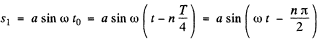Thus the sought distance covered for odd n is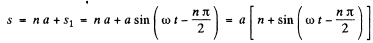Case (2), when n is even, In this case from the equation x = a cos ωt, the distance covered ( s2 ) in the interval t0, is given by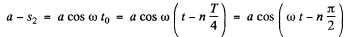or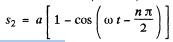Hence the sought distance for n is even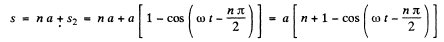In general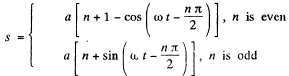Q. 8. At the moment t = 0 a particle starts moving along the x axis so that its velocity projection varies as v = 35 cos πt cm/s, where t is expressed in seconds. Find the distance that this particle covers during t = 2.80 s after the start.

Ans. Obviously the motion law is of the from, x = a shoot, and vx = ω a cos ωt.
Comparing vx - ω a cos ωt with vx - 35 cos πt , we get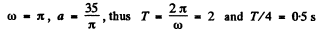Now we can write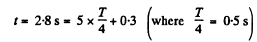As n = 5 is odd, like (4 = 7), we have to basically find the distance covered by the particle starting from the extreme position in the time interval 0 = 3 s.
Thus from the Eqn.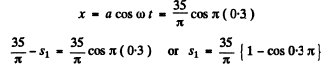Hence the sought distance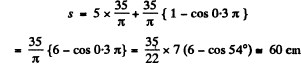Q. 9. A particle performs harmonic oscillations along the x axis according to the law x = a cos ωt. Assuming the probability P of the particle to fall within an interval from —a to +a to be equal to unity, find how the probability density dP/dx depends on x. Here dP denotes the probability of the particle falling within an interval from x to x dx. Plot dP/dx as a function of x.

Ans.  As the motion is periodic the particle repeatedly passes through any given region in the range - a ≤ x ≤ a.

The probability that it lies in the range (x, x + dx) is defined as the fraction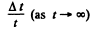where Δt is the time that the particle lies in the range (x, x + dx) out of the total time t. Because of periodicity this is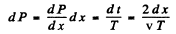where the factor 2 is needed to take account of the fact that the particle is in the range ( x , x + d x ) during both up and down phases of its motion. Now in a harmonic oscillator.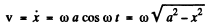Thus since ωT = 2 π ( T is the time period)

We get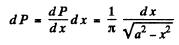Note that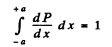so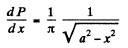Q. 10. Using graphical means, find an amplitude a of oscillations resulting from the superposition of the following oscillations of the same direction:
(a) x =3 .0 cos (ωt -1- π/3), x2  =8 .0 sin (ωt + π/6);
(b) x1 =  3.0 cos ωt, x2  = 5.0 cos (ωt+ π/4), x3  =6 .0 sin ωt.

Ans.  (a) We take a graph paper and choose an axis (X - axis) and an origin. Draw a vector of magnitude 3 inclined at an angle π/3 with the X -axis. Draw another vector of magnitude 8 inclined at an angle - π/3( Since sin (ωt+ π/ 6 ) » cos (ωt- π/ 3 )) with the X - axis. The magnitude of the resultant of both these vectors (drawn from the origin) obtained using parallelogram law is the resultant, amplitude.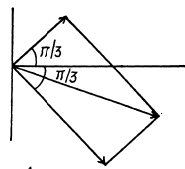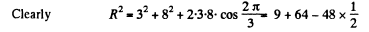Thus            R = R7 units

(b) One can follow the same graphical method here but the result can be obtained more quickly by breaking into sines and cosines and adding :
ResultantThen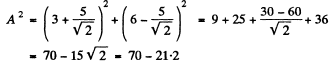So,   A = 6-985  = 7 units
Note- In using graphical method convert all oscillations to either sines or cosines but do not use both.

Offer running on EduRev: Apply code STAYHOME200 to get INR 200 off on our premium plan EduRev Infinity!

111 docs

,

,

,

,

,

,

,

,

,

,

,

,

,

,

,

,

,

,

,

,

,

,

,

,

;